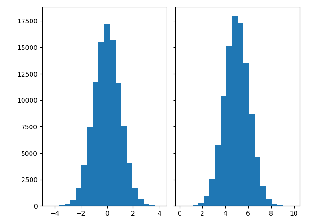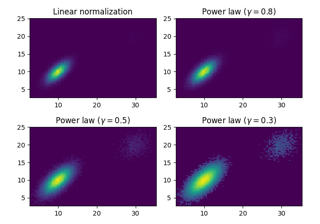# matplotlib.axes.Axes.hist2d¶

Axes.hist2d(self, x, y, bins=10, range=None, density=False, weights=None, cmin=None, cmax=None, *, data=None, **kwargs)[source]

Make a 2D histogram plot.

Parameters: x, yarray-like, shape (n, )Input values binsNone or int or [int, int] or array-like or [array, array]The bin specification: If int, the number of bins for the two dimensions (nx=ny=bins). If [int, int], the number of bins in each dimension (nx, ny = bins). If array-like, the bin edges for the two dimensions (x_edges=y_edges=bins). If [array, array], the bin edges in each dimension (x_edges, y_edges = bins). The default value is 10. rangearray-like shape(2, 2), optionalThe leftmost and rightmost edges of the bins along each dimension (if not specified explicitly in the bins parameters): [[xmin, xmax], [ymin, ymax]]. All values outside of this range will be considered outliers and not tallied in the histogram. densitybool, default: FalseNormalize histogram. See the documentation for the density parameter of hist for more details. weightsarray-like, shape (n, ), optionalAn array of values w_i weighing each sample (x_i, y_i). cmin, cmaxfloat, default: NoneAll bins that has count less than cmin or more than cmax will not be displayed (set to NaN before passing to imshow) and these count values in the return value count histogram will also be set to nan upon return. h2D arrayThe bi-dimensional histogram of samples x and y. Values in x are histogrammed along the first dimension and values in y are histogrammed along the second dimension. xedges1D arrayThe bin edges along the x axis. yedges1D arrayThe bin edges along the y axis. imageQuadMesh cmapColormap or str, optionalA colors.Colormap instance. If not set, use rc settings. normNormalize, optionalA colors.Normalize instance is used to scale luminance data to [0, 1]. If not set, defaults to colors.Normalize(). vmin/vmaxNone or scalar, optionalArguments passed to the Normalize instance. alpha0 <= scalar <= 1 or None, optionalThe alpha blending value. **kwargsAdditional parameters are passed along to the pcolormesh method and QuadMesh constructor.

hist
1D histogram plotting

Notes

• Currently hist2d calculates its own axis limits, and any limits previously set are ignored.
• Rendering the histogram with a logarithmic color scale is accomplished by passing a colors.LogNorm instance to the norm keyword argument. Likewise, power-law normalization (similar in effect to gamma correction) can be accomplished with colors.PowerNorm.

Note

In addition to the above described arguments, this function can take a data keyword argument. If such a data argument is given, the following arguments can also be string s, which is interpreted as data[s] (unless this raises an exception): x, y, weights.

Objects passed as data must support item access (data[s]) and membership test (s in data).

## Examples using matplotlib.axes.Axes.hist2d¶